1.800.221.5175
Core Program
Full Access
Core Program
Full Access
High-Impact Tutoring
Core Program
Full Access
National Program
Full Access
New York
Critical Thinking for Active Math Minds
Preparing for Standards Based Assessments
Vocabulary Workshop, Tools for Comprehension Grades 1–5
Print Program
Interactive Edition
Print Program
Interactive Edition
Vocabulary Workshop, Tools for Excellence Grades 6–12+
Print Program
Interactive Edition
Vocabulary for Success
Progress English Language Arts Grades K–8
National Program
Full Access
High-Impact Tutoring
New York
Grammar Workshop, Tools for Writing
Grammar for Writing
Print Program
Interactive Practice Bundle
Fluency Booster Practice Book
Interactive Assessments
High-Impact Tutoring
Building Reading Success with Wiley Blevins
Print Program
Interactive Edition
Professional Learning

## How Math Games and Activities Increase Student Proficiency in Grade 6

Math games and activities can be an excellent tool to supplement and support math instruction. Using math games in your classroom allow students to practice mathematics in fun but also impactful ways. Students love games because they are engaging and exciting, and teachers love games because they help students practice what they've learned.

Grade 6 instruction is focused on ratios, fractions, statistical thinking, and using variables in mathematical expressions. These topics are facilitated by practice with the following items for enrichment: diagrams in algebraic problem solving, simple geometric models, demonstrating proportional thinking graphically, and reconstructing the fraction division algorithm.

## Geometric Model

for Variable Expression

### Geometry helps students visualize the role that a variable expression can play. Say that a designer decides to keep a rule for each rectangular cut-out that she makes:

“The length is always 3 inches longer than the width.” Present this table: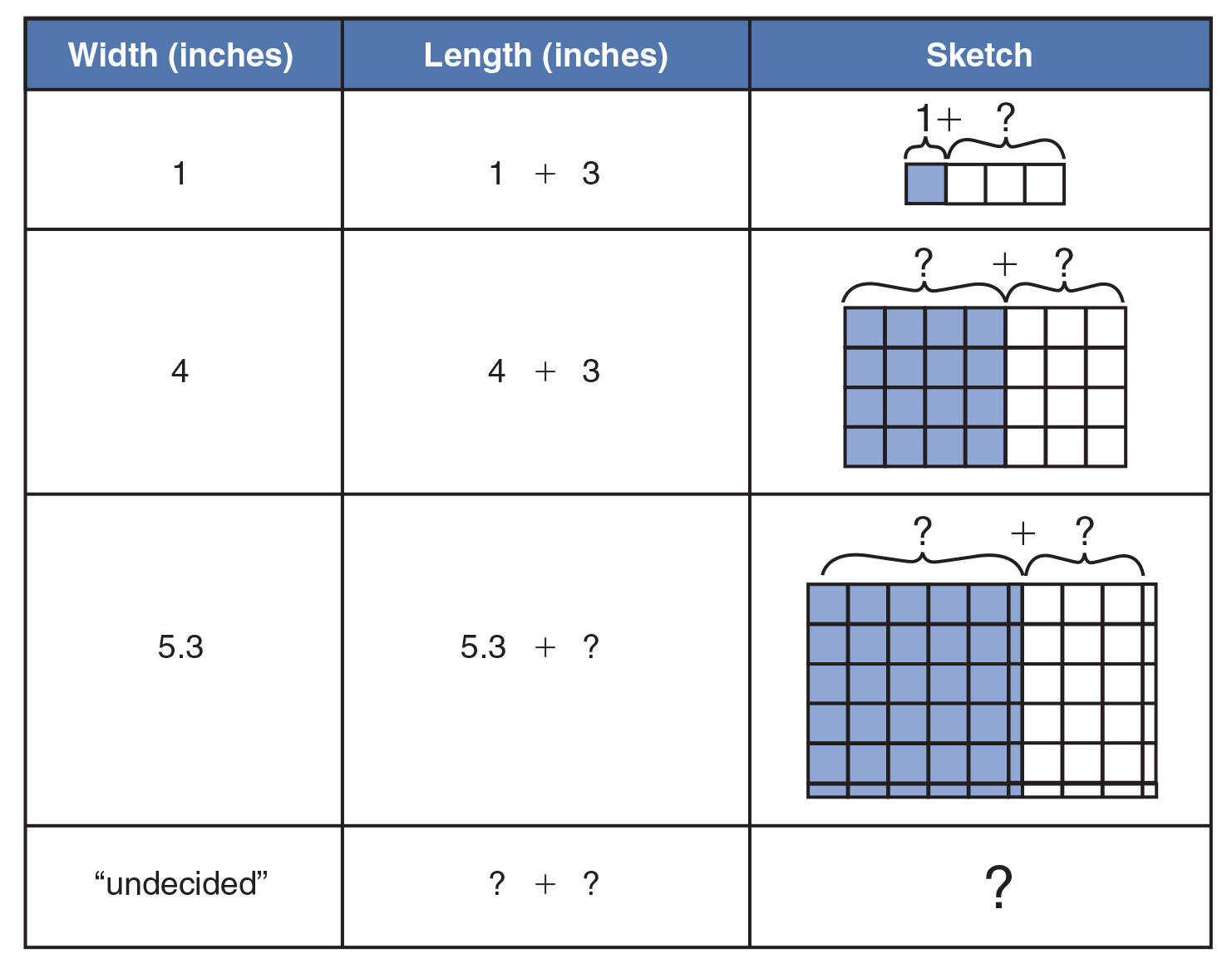Have the class fill in the question marks for the sketches in the first two rows. Ask: “What is the same and what different between these two rows?” (Width changes or varies; length is always the width plus an unchanging or constant amount, 3). In the third row have students provide the missing values, as well as the rule by which they did so:

“Add 3 to the width” or the equivalent. In the last row point out that the designer may not know what width to choose next, or may put her work aside, but that the rule remains the same. Ask for ideas on replacing the question marks with words. Accept all equivalent answers, such as:

• “3 added to some width”
• “Whatever, plus 3”
• “‘We’ll see’ + 3”

as well as ideas for symbols for the unknown width, such as ☐, X, w, etc. Stress that all these responses are algebraic expressions, whether they include a word, sign, letter, and that the part that stands for the changeable, or varying, amount is a variable. All choices are valid; a buzz or other sound, a color or hand signal could serve as well, provided we agreed what the symbol stood for—a whole class of number possibilities.

Now enrich the model as follows, having students work in groups to make their own pictorial models and symbolic substitutions: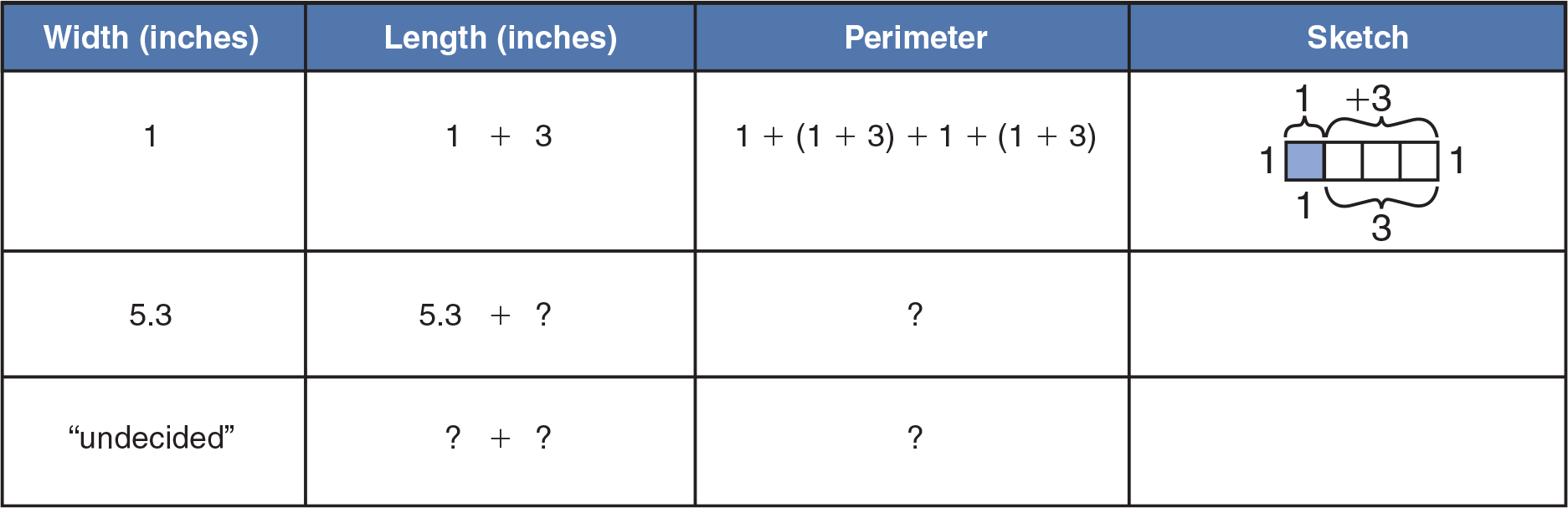Student responses will lead to the observation that the rule or expression for perimeter can involve different operations and different organizing and ordering symbols, such as parentheses. For the perimeter expression, students may offer

2 × [ (undecided width + (undecided width + 3) ]

besides many other acceptable versions of varying formality and abstractness.

References
“Establishing Fundamental Concepts through Numerical Problem Solving,” F. Demana & J. Leitzel of Ohio State University, in The Ideas of Algebra, NCTM, 1988 Yearbook

## Building the Function Concept

from Raw Data Up

### Students may well question the importance of 2-variable equations when the function rule is presented as an abstraction, without context, from which solutions are deduced using only math rules. In practice, engineers and scientists often work in the opposite sense, building equations up from empirical solutions, or patterns of values, given them by nature. This activity shows that an equation can tell even more about a physical situation than the data patterns alone.

Explain that we often do not have a rule for how one factor in nature affects another. For example, how does the weight on a spring change the spring’s length? Have students think of the springs under their bike or car seats. What if a mechanic put in the wrong spring—one that stretched too little or too much?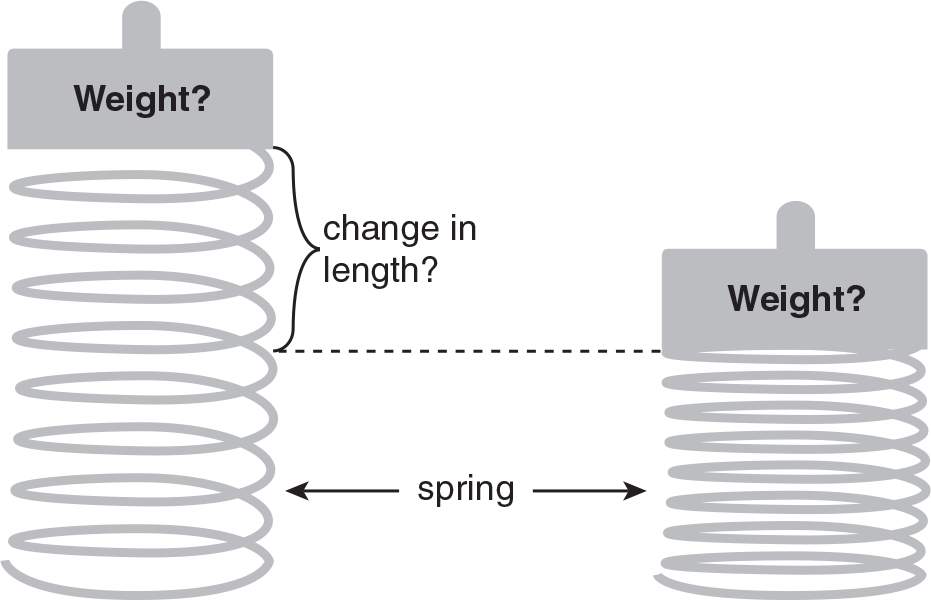The table relates the weights on a spring and the spring’s reduction in length. Challenge students to generalize from the data to a rule that matches the data. Accept responses of varying formality (some appropriate ones are given).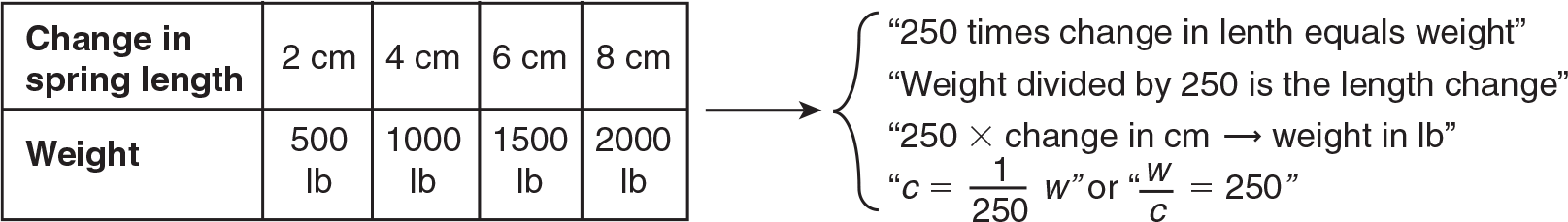Let students working in groups graph the weight-length change relation. Discuss whether they should use values from the table, or new values generated by the math rule they discovered (either or both; they all describe the same situation).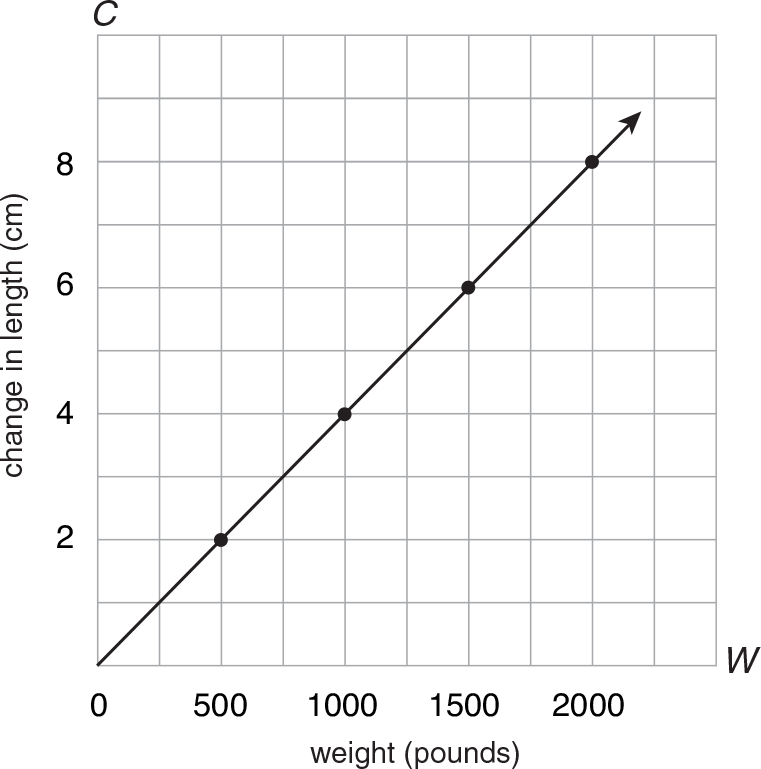Now guide your students to discover all that the graph can tell us:

• What always happens when the weight increases? (change in length increases)
When the weight decreases? (change in length decreases)
• What do you predict about the weight on the spring if the change in length was very small? (small change in weight) If the change in length were zero? (no change in weight)
• What would the change in length be for 1250 lb? (5 cm) How do you know? (compute from the equation C = W/250 or move from 1250 on W-axis up to solution line and then across to value 5 on C-axis)
• How many number pairs, or solutions, does the table show? (4) How many do the graphs show? (an infinite number) Including fractional values, or just whole numbers? (fractional numbers, too) A value of W for any value of C? (yes) A value of C for any value of W? (yes)

## Coins as a Model of Division

Decimals

### The division of decimals by decimal fractions—that is, by divisors that may be smaller than the dividend—yield a quotient greater than the dividend. One source of student difficulties at this stage is a mental model, still rooted in the partitive concept, by which the object of division (dividend) is partitioned or “meted out” into smaller, equal parts: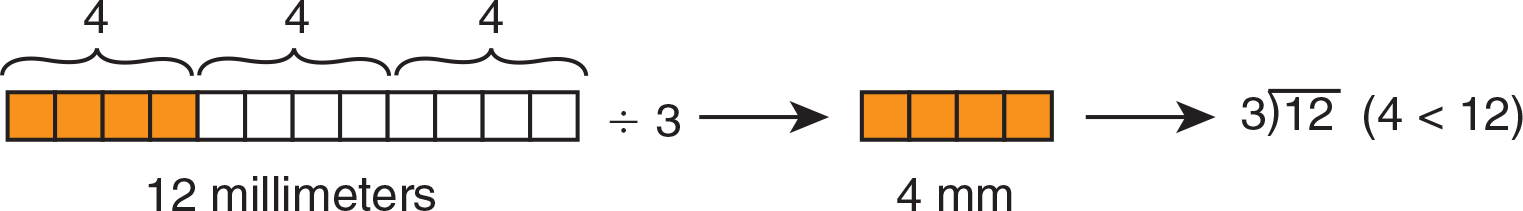This not always apt model may persist through the teaching of division of decimals: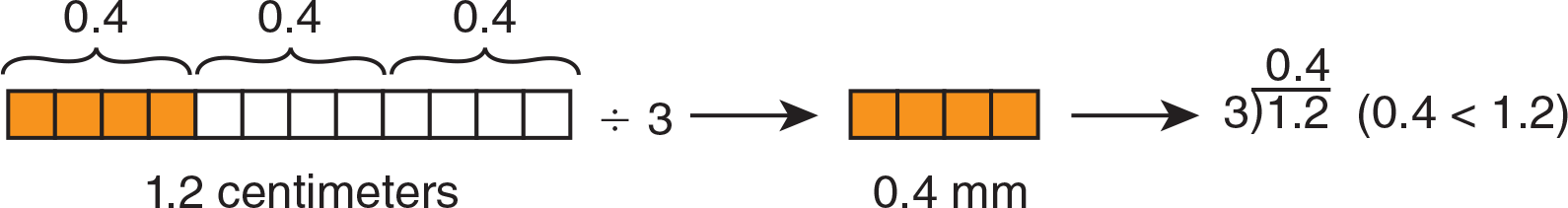Note the expectation in both cases: the quotient must turn out greater than the dividend. Upon the introduction of division by decimal fractions, the student is handed an algorithm that gives a markedly different result: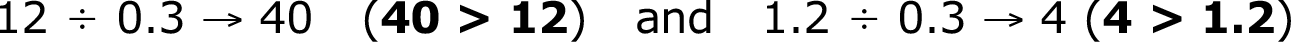These quotients are hard to verify or predict by number sense and not readily shown pictorially as partition. (What is meant by, “Separate it into 0.3 equal pieces”?) Rather, the question should become: How many lengths of 0.3 “go into” the total length?

Money is an excellent medium by which to model the idea of division by fractional divisors and quotients greater than dividends (and to counter older and often confused models). Because money is denominated in units such as nickels and dimes that are at the same time decimal fractions of a dollar, coin problems are ready-made to give students an intuitive feel for the proper magnitude of the outcome. Distribute play coins to groups, posing questions such as the following, and demonstrating solutions.

(1) How many nickels in two dimes?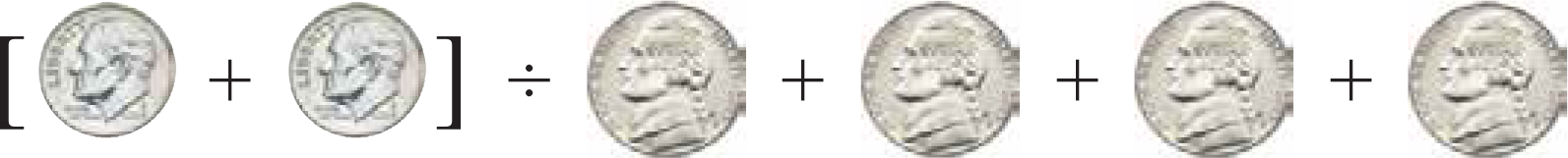(2) How many nickels in a quarter?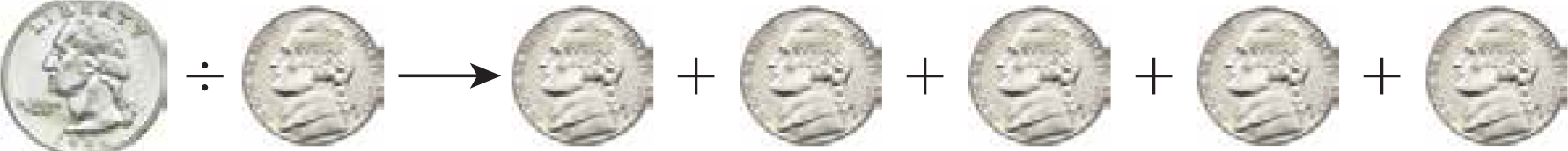(3) How many quarters in a half-dollar?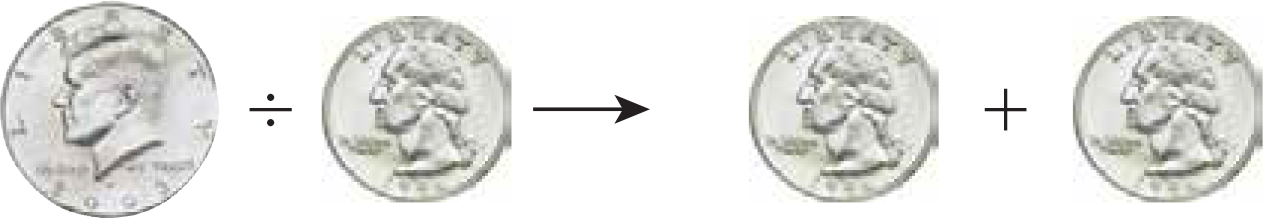For each manipulative demonstration, have student volunteers represent (and explain in their own words) the symbolic calculation:Stress that the answer (quotient) is greater than the amount to be divided (dividend) because the problem asks how many small—or fractional—parts are in a large part. Point out that this is why a division exercise like 4.396 ÷ 0.28 has a quotient of the magnitude 15.7—much larger than either divisor or dividend. Approximate such a “complicated” long division using compatible numbers:So the answer, 15.7 ≈ 16, makes number sense.

References
“Multiplication and Division: From Whole Numbers to Rational Numbers”, A. O. Graeber and E. Tanenhaus, in Research Ideas for the Classroom, MacMillan/NCTM, 1993

### Here are tips on using color counters to model the various cases of adding and subtracting. These procedures are more “stepped out” than the relatively abstract rules in the text. Have students consistently use two colors: for example, blue for negatives and red for positives.

Adding Integers with Like Signs: –6 + –1 = ?

These are simple. Add up the counters and the color tells the sign.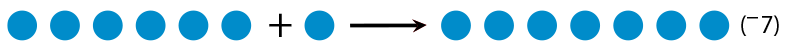Adding Integers with Unlike Signs: +2 + –5 = ?

In these cases students can cross out “zero pairs,” which have zero value.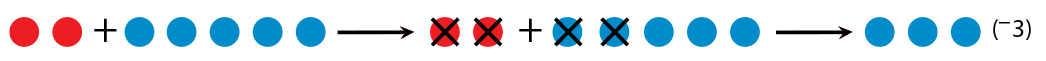Subtracting Integers with Like Signs: –3 − –2 = ?

To subtract like signs, students simply “take away.”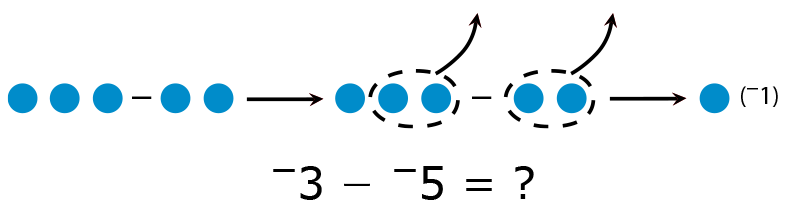However, if the number to be subtracted (–5) has the greater absolute value, students need to create zero pairs to subtract (take enough away).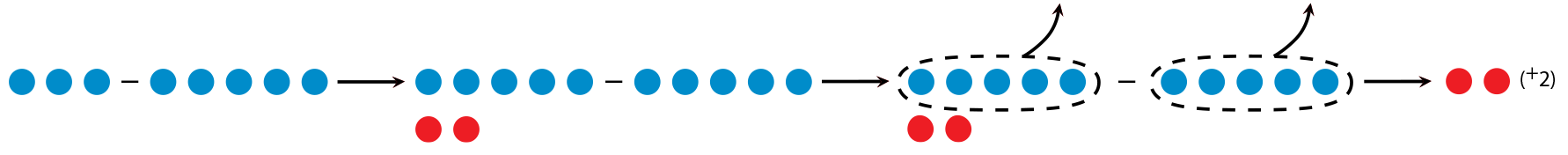Subtracting Integers with Unlike Signs: –4 − +3 = ?

With unlike signs, zero pairs need to be created. Emphasize to students that zero pairs have zero value, so creating them does not change the answer, it only lets us “take away.”+1 − –4 = ?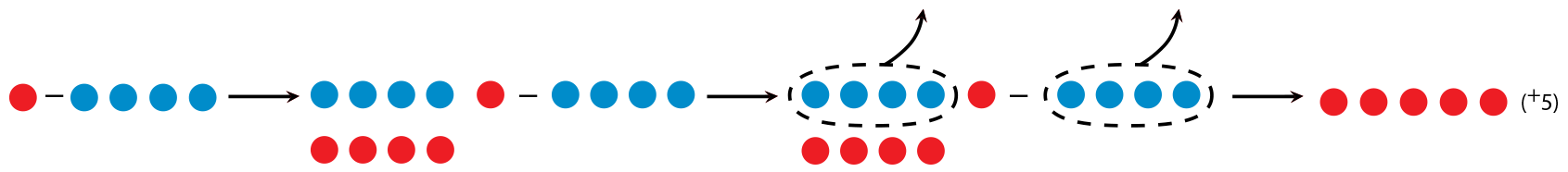Have students verbalize the “what’s and why’s” of each step as you go along.

1 "Fractional Numbers," from Teaching Elementary School Mathematics for Understanding, Marks, Purdy, et al, McGraw-Hill, 1975

## Proportional Thinking

Shown Graphically

### Proportionality is a rich idea that involves co-variation of factors (or multiple comparisons), thus requiring the storing and processing of several bits of information simultaneously. The teaching of proportions is traditionally embedded in missing term problems, which define proportion as a statement that two ratios are equal:

a/b = c/d

But students soon find that the idea extends beyond this to mean:

y/x = constant or y = constant • x

that is, that an infinite number of equivalent ratios may be found for any given ratio. To provide a graphical interpretation of both these aspects of proportion, it is not necessary to use ordered pairs formally; your students have encountered a coordinate system of relationships at earlier levels as line graphs and simplified grids or maps. Present this problem along with the coordinate grid below:

Sally pays 6 dollars for 2 mini-pizzas. At that rate,
how much will she need to pay for 3 mini-pizzas?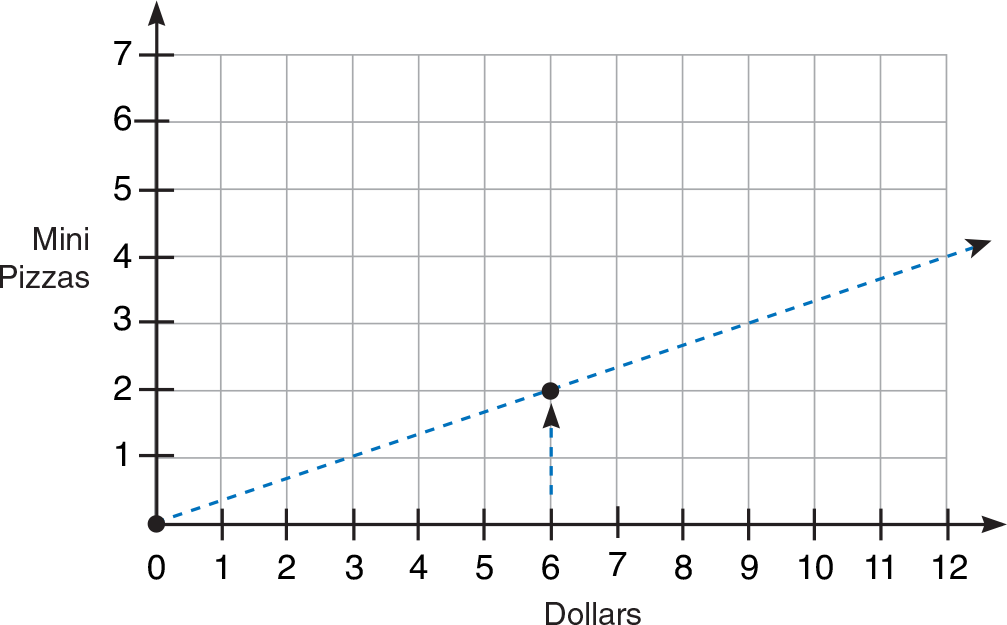Explain that the grid has 2 points marked for the 2 facts already known: 2 pizzas for 6 dollars, and 0 pizzas (of course) for 0 dollars. Join the points with a straight line. Extend the line beyond, explaining that the same rate, or cost per pizza, applies to any number of pizzas. Have a volunteer find the cost for 3 mini-pizzas on the graph, moving horizontally from 3 on the pizza axis to the line, and down vertically to \$9 on the dollar axis. Point out that the graph shows the proportions

dollars/pizzas = 62           dollars/pizzas = 93            62 = 93

An advantage of the graphical model is that any equivalent ratio may be found—that is, the solution to any problem based on the same cost per pizza—including the unit rate. Now have students use the graph to solve for:

• the cost of 4 mini-pizzas (12 dollars)
• how many mini-pizzas you can get for \$15 (five); for \$15 million (5 million)
• the unit rate for mini-pizzas (\$3 per pizza)
• the amount you owe a friend for eating 12 of their pizza (\$1.50)

Pointing to any point on the line, ask what students know about that point (it shows the same rate of dollars to pizzas, 3 to 1). You may point out that the relationship can also be represented as

dollars/pizzas = 31           or            dollars = 3 × pizzas

Explain that this statement is always true and that, in the equation on the right, the 3 is called a constant of proportionality, because the 3 remains the same, regardless of how many pizzas are bought.

References
Van de Walle, J. A. (1998). Elementary and Middle School Mathematics: Teaching Developmentally, 55, 401 New York, NY
Proportionality and the Development of Pre-Algebra Understandings,” The Ideas of Algebra, NCTM Yearbook, T. R. Post, et al, 1998

## Diagrams in Algebraic Problem Solving

### Students sometimes struggle in algebra because of their earlier, unsuccessful attempts to solve problems with variables. Social interest problems can sell students on the power of algebra, but they may need guidance to strengthen their commitment. By using diagrams, you help students access their own reason using representations that fall between words and algebraic symbolism.

Present this problem:
Jen invited her whole class, which was 35 girls, to a dance. To make equal numbers of boys and girls, she also invited, from her neighborhood, the same number of boys as in class, plus 6 more girls. How many kids are in Jen’s class?

Suggest starting off with a diagram of the class and what is known about it.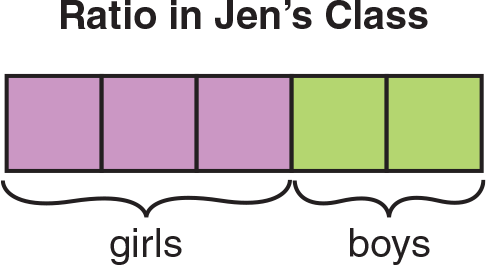Students will see that this is not enough information. Challenge volunteers to add to the diagram to show what happens next. Use prompts, if necessary, like:

• How could you show double the number of boys?
• How could you show that 6 more girls were invited?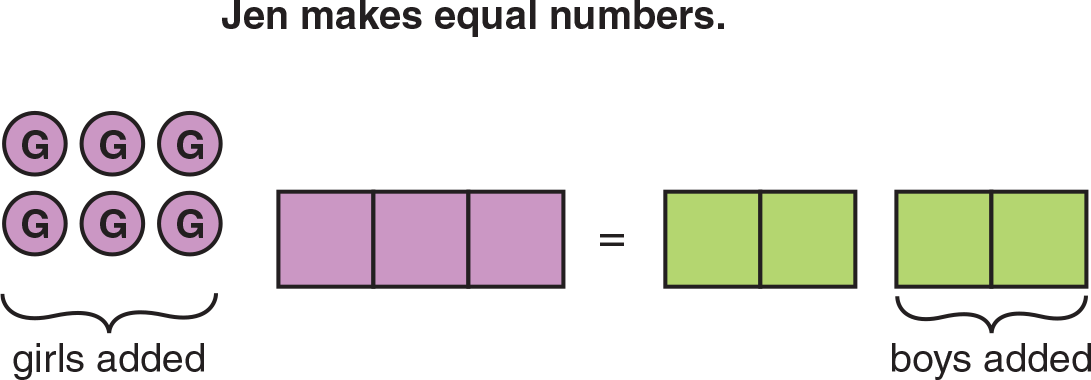• How does the situation in the class change once the other kids are added? (The number of boys and girls is now equal.) How can you show this in the diagram? (with an equals sign)

Continue the prompting, if necessary:

• Does each box stand for the same number of boys or girls? (yes) How many? (unknown, but the same number, since each box is 15 of the whole class number)
• How can you find how many in each box?

Here are some creative responses to look for:

• Since the boxes are equal and there are the same number of boys and girls, there should be the same number of boxes for girls as boys—4 boxes each.

You might enclose the 6 extra girls in a box at this point.

• There must be 6 girls in each of the 3 boxes, and 6 boys in each of the other 4 boxes.
• 4 boxes of boys equal 3 boxes of girls + 6. So there must be 6 in a box.
• 4 × 􏰄 = 3 × 􏰄 + 6 → (4 × 􏰄) − (3 × 􏰄) = 6 → 1 × 􏰄 = 6

Have students give the solution: 5 boxes of 6, or 30 boys and girls altogether. You may want to go back to the diagrams to show the step-by-step process. Only the first two steps are given here:

• 3k + 2k = c ← Set up variables for equal groups of boys and girls and for the class, based on the fractions of 35 and 25.
• 3k + 6 = 4k ← 3 groups of girls plus 6 equals 4 groups of boys.

References
Simon, M., and Stimpson, V. “Developing Algebraic Representation Using Diagrams” In The Ideas of Algebra, K–12: 1988
Yearbook of the National Council of the Teachers of Mathematics, edited by Albert P. Shulte and Arthur F. Coxford, 136–41. Reston, VA: NCTM, 1988.

## Fractions: From Area to Symbolization

### Distribute squares and have students shade regions to demonstrate 1⁄2. Look for a variety of correct solutions, such as:Ask questions such as the following:

• Do all the shaded parts have the same shape? (No)
• Is the same number of parts shaded? (No)
• Is the same amount of area shaded? (Yes)
• How does the number of shadeed parts compare to all parts? (1 to 2 or 2 to 4 or 4 to 8)
• Then how do you know the ratio or comparison is the same—one-half? (You can multiply one term by 2 to get the other term; or The ratios all equal 12.)

Distribute templates paritioned as below, and ask students to model 12.• Does 12 have one shaded part and two parts in all? (Not necessarily) One shaded part for each unshaded? (Yes)
• What is the comparison of shaded to all parts in these pictures? (4 to 8)
• How can that ratio mean "one-half"? (There are 2 times as many parts in all than shaded parts; or There are 12 as many shaded than in all; or 4 to 8 equals 1 to 2.)
• What names are there for this fraction? (12 or 48) Why are these fractions the same number? (They compare the same areas.)
• How did you know how many parts to shade? (Match one shaded for one unshaded; or Divide 8 by 2 to get 4; or One-half of 8 is 4.)

On a third template, have students represent and symbolize fractions as they please.
For example: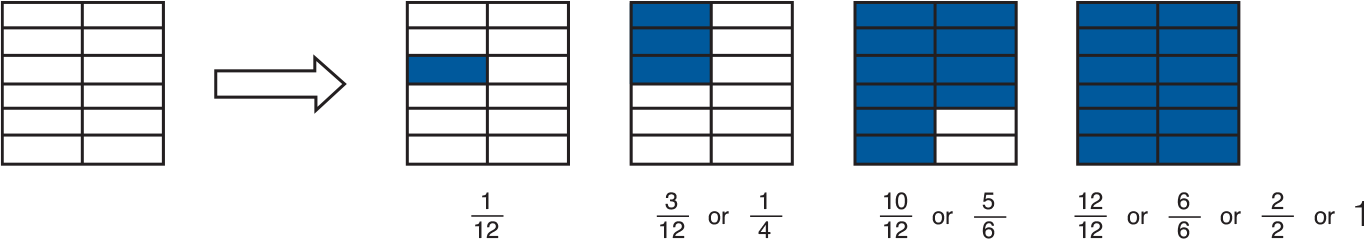• How are these fractions the same, and how are they different? (There are 12 parts in all, but the same number is not always shaded.)
• How can all the same fractions have different names? (The different names represent equivalent fractions; or The areas shaded are in the same ratio.)

1 The activity on this page is based on: "Fractional Numbers," from Teaching Elementary School Mathematics for Understanding, Marks, Purdy, et al, McGraw-Hill, 1975

## Introducing "Proof" in Geometry

### All students profit from actively deriving a more abstract and general truth from simpler elements acquired earlier. The following activity exemplifies the process by which geometric knowledge is synthesized, using “proof” formulated at an appropriate level of formality.

Have volunteers cut out and display a variety of triangles. Ask the class whether

• these triangles all have congruent corresponding angles (no).
• the sums of the angle measures in all are equal (students may or may not recall that the sum of the angle measures equals 180).

Let students demonstrate the apparent truth of this fact by tearing off 2 corners of each triangle to form a straight angle: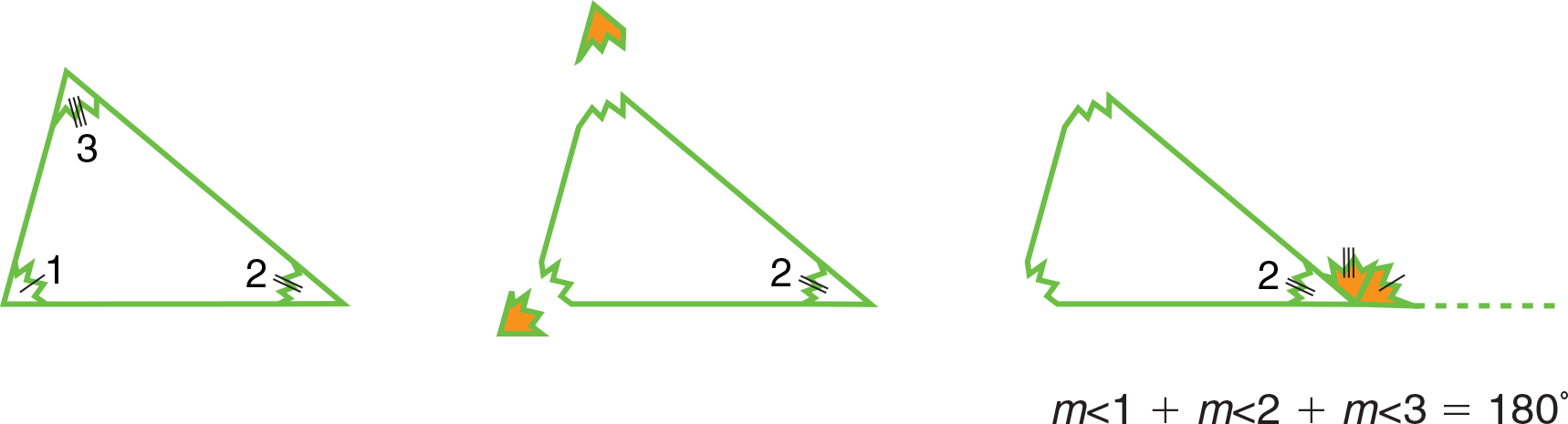Ask whether this property is true of every triangle (yes; explanations will vary). Point out that short of testing every triangle in the world, we could not know this for certain, or why this should be the case. Suggest that you are looking for a demonstration as clear as tearing corners, but convincing for all shapes of triangles, seen and unseen. Present parallel lines and a transversal, as shown: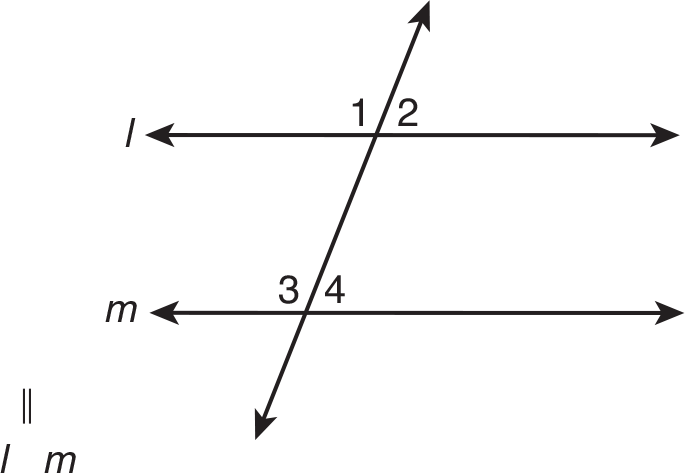Have students recall how the interior angles relate (m∠1 = m∠3 and m∠2 = m∠4). Add a 2nd transversal (t), just as shown, with re-labeled angles.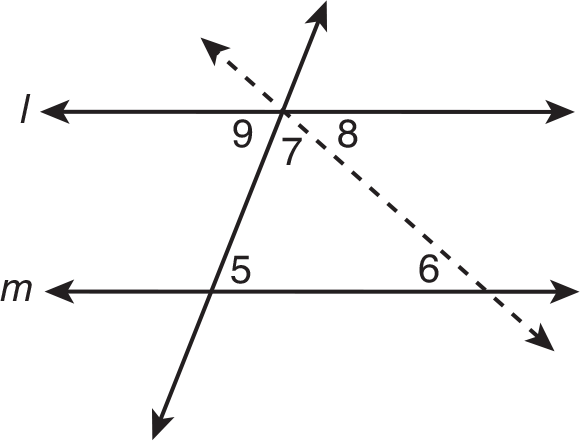Challenge the class to show that the newly formed angles 5, 6 and 7 have a sum of 180. Let them grapple with the problem, even if their insights are hesitant, at times off target or poorly expressed. Have students independently record their ideas at their desks, then let volunteers give their rationales. Processes of thinking will vary but include the following:

• ∠9 + ∠7 + ∠8 is a straight angle (measures equal 180).
• m∠9 = m∠5 and m∠8 = m∠6
• You can substitute ∠5 and ∠6 into the first equation to get m∠5 + m∠7 + m∠6 = 180.

Ask whether this must be true for every triangle in the world (yes, since any shape of triangle can be formed by the 2nd transversal). Explain that the class has constructed on its own a geometric proof of the kind first developed by Euclid 2,300 years ago.

## Modeling the Mean for Understanding

### In everyday life, processing statistical data depends on a few basic measures, such as the mean. Though it is simple to calculate, even many adults do not fully understand the mean—a single number that represents a whole pool of data and communicates several ideas about that pool. These activities are designed to ensure that your students grasp the key properties of the mean.

(A) Zero as the Sum of the Deviations from the Mean
This property implies that all data of a set “balance out” in two directions to form the mean. When estimating a mean, or assessing how adding a datum to a set affects the mean, this property is critical. Present the “balance” model below, and have students construct various data sets to match the given mean (30).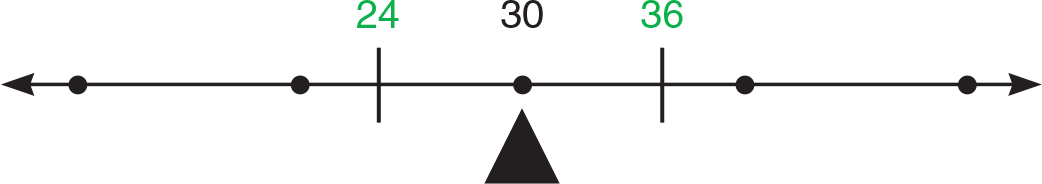Suggest at first a small set of even-numbered elements; in response, students might give 24 and 36. Listen carefully to students’ reasoning. For example:

• “36 is 6 more than 30, so it can be paired with a number 6 less than 30”.
• “6 in one direction plus 6 in the other direction means zero change—so the mean stays 30”.

Have the class use more numbers, as well as odd-numbered elements, when building sets with a given mean. Be sure students realize that all their sets have a common property: the sum of the differences from the mean is zero.

(B) Effect of Zero on the Mean
Many think that inclusion of zero in a set has no impact on mean. To counter this error, present the balance and a mean at the fulcrum, with students providing the set. Then have students show the effect of adding a zero to the model. It will be evident that the zero datum “weighs down” the mean in one direction, which can be offset by another datum, in the opposite direction, of equal difference to the mean.

(C) The Mean Stands for All Data, but Is Not Necessarily Among the Data
Many who compute the mean easily do not grasp that it stands for the entire set; often, not being itself among the group, it is difficult to visualize. Starting with a set of 2 given data points, then progressing in complexity, have students place the fulcrum point (the mean) on the number line: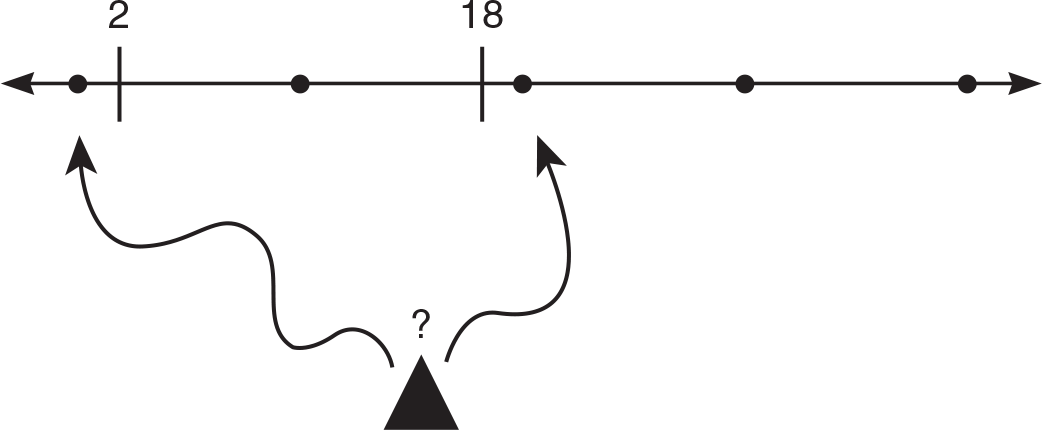Finally, using the models, pose questions to verify that students understand that:

• there is one and only one mean for a given set of data.
• different sets of data can have the same mean.

References
Bright, G. W., and Hoeffner, K. “Measurement, Probability, Statistics, and Graphing.” In Middle Grades Mathematics: Research Ideas for the Classroom, edited by D. T. Owens, 41–57. New York: Simon & Schuster Macmillan/NCTM Research Interpretation Project, 1993.

## Number Lines to Add and Subtract Fractions

### Research tells us that students—even those in junior college—do not typically respond to iconic fraction models that have previously failed them1. One strategy is to use less common models, including such relatively abstract ones as number lines, to ensure that students approach the topic fresh, without mental blocks. Students thus start not with the aim of finding LCMs; instead, given number lines with equivalent fractions, they choose those that let them compute most readily. The patterns that emerge suggest to students the “rules” generally required.

Present this number line: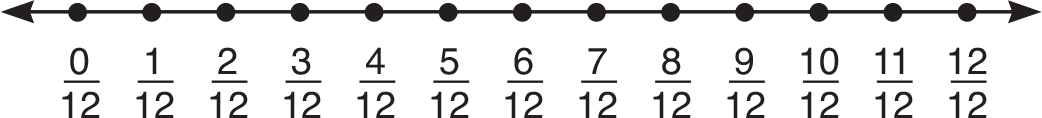Have students add and subtract these fraction pairs mentally, then ask the questions that follow:• How did you find the answers? (Add/subtract the top term, or numerator.)
• Why are these problems so easy? (All the fractions are parts of a whole with the same bottom term, or denominator.)

Now show this pair of number lines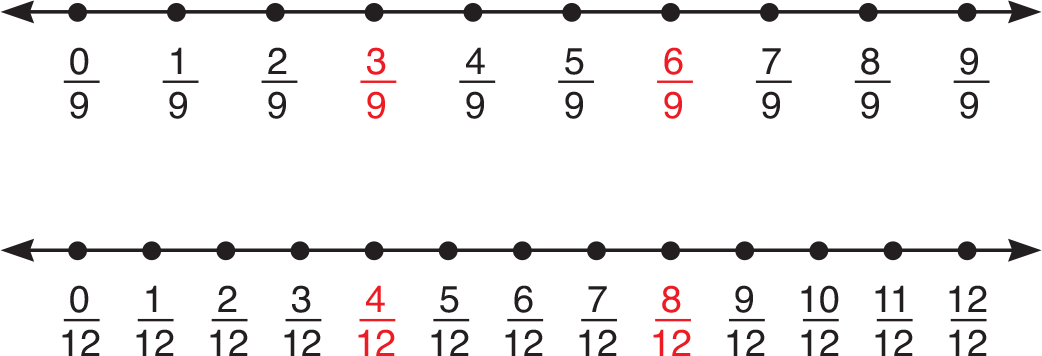and have students evaluate these expressions mentally:• How did you do this without paper and pencil? (These fractions represent the same 13 points on two number lines—either in 19's or in 312's.)

Have students try these (which do not have common points), again mentally: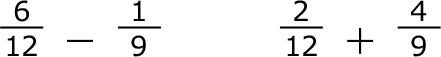• What is the difficulty? (None of these fractions are given as the same points, or equivalents.) What to do? (Try other number lines.)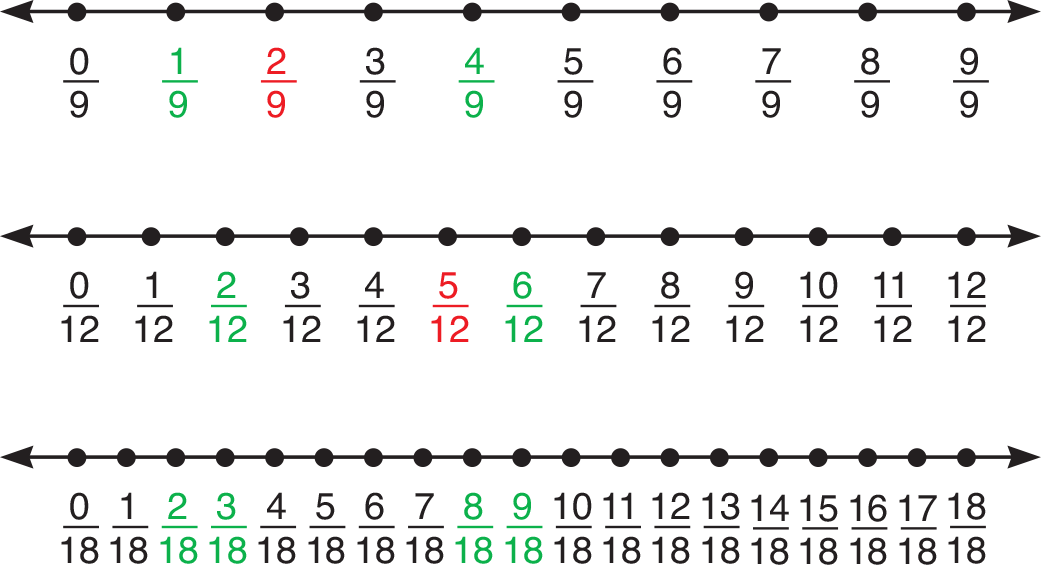Now those answers are visually accessible: 61219 = 718, 212 + 49 = 1118.

• How could you find 29 + 512? (There are no common points for them on these 3 number lines; you would need another number line.)
• How could we figure out which number line(s) we would need? (Possible answers: It could be in 136's, since 19's and 112's are made up of 136's or It could be 136's, since 36 is divisible by 9 and 12.)

References
Teschner, G. Math Mastery Series: Fractions, Part 1. Elizabethtown, PA: Continental Press, 1989. 1 National Association for the Education of Young Children, (1999). Mathematics in the Early Years, 54

## Fraction Division Algorithm

### Operating with fractions can be an obstacle to success at the middle, high school, and even junior college levels. In particular students choose between the division and multiplication algorithms incorrectly. This activity shows an easy technique for retrieving the algorithms at any time and deciding quickly which to use.

Tell students it is easy to decide when to multiply by the inverse, whether they forget the division procedure or confuse it with the multiplication procedure. Show this number line. Have students estimate, without paper and pencil, the quotient.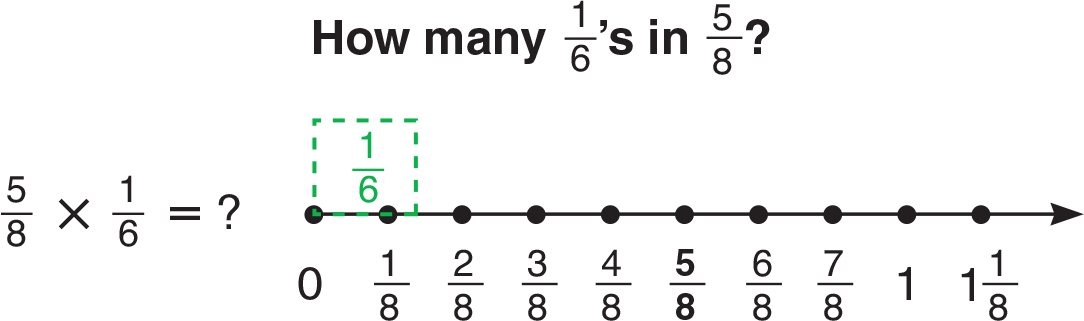It is visually clear the quotient is somewhat less than 5. Now give the standard algorithms for multiplying and dividing, without saying which is which: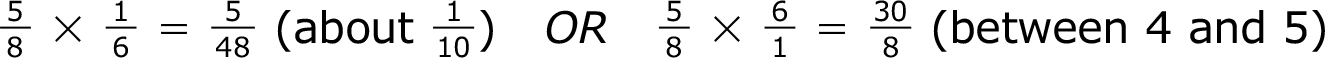Have students tell you which must be for dividing. (The one at right, where you multiply by the reciprocal of the divisor.) Present the next number line and a slightly harder division, where the divisor exceeds the dividend. Have a volunteer sketch in for the others the approximate size of the dividend, 13: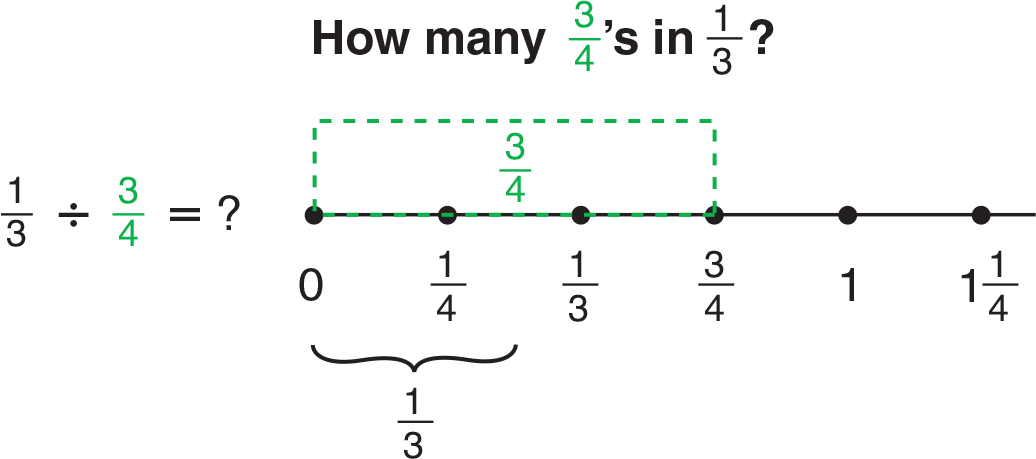It can be seen that there is a little less than one-half of 34 in 13. Again, have the class choose the right algorithm (the one at left, where the reciprocal is used).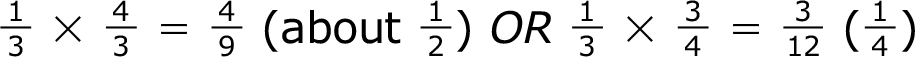Simple “checks” like these make it unnecessary to worry about the reciprocal rule while solving problems or during a test. Point out that it is easy and fast to reconstruct what you need to do:

• Jot down a problem you can do in your head; for example, 23 ÷ 13 = 2
• If helpful, model the problem: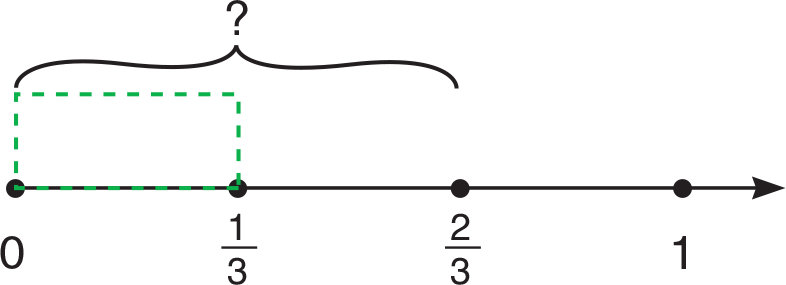• Write out the algorithms you are not sure of. Which matches?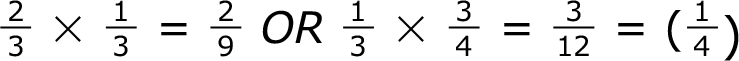This technique takes up much less time and effort than hesitating or re-working problems. For students who want to see why the division algorithm works, share the arithmetic demonstration on the opposite page.

References
Van de Walle, J. A. (1998). Elementary and Middle School Mathematics: Teaching Developmentally, 55, 401 New York, NY

## Money Model and Decimal Multiplication

### Students will easily visualize the purpose and meaning of decimal multiplication in a demonstration of money computation. Present the mixed decimal value 1.52 as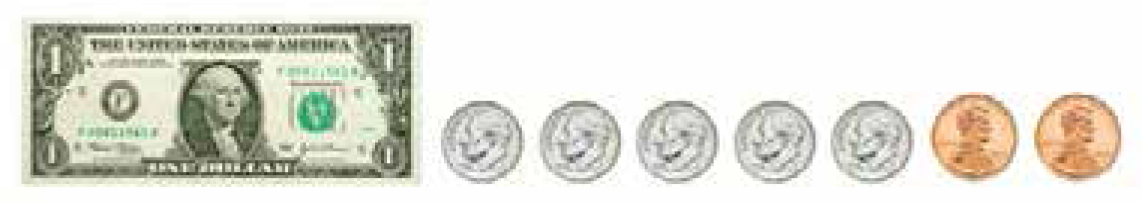Be sure to verify that students understand that the pictorial quantity is properly under- stood in alternative ways: either as 152 pennies or as 1 and 52/100 (0.52) dollars.

Now suppose that you were to find how much three times that amount would be. Repre- sent the result as the simple repetition, three times, of the model above.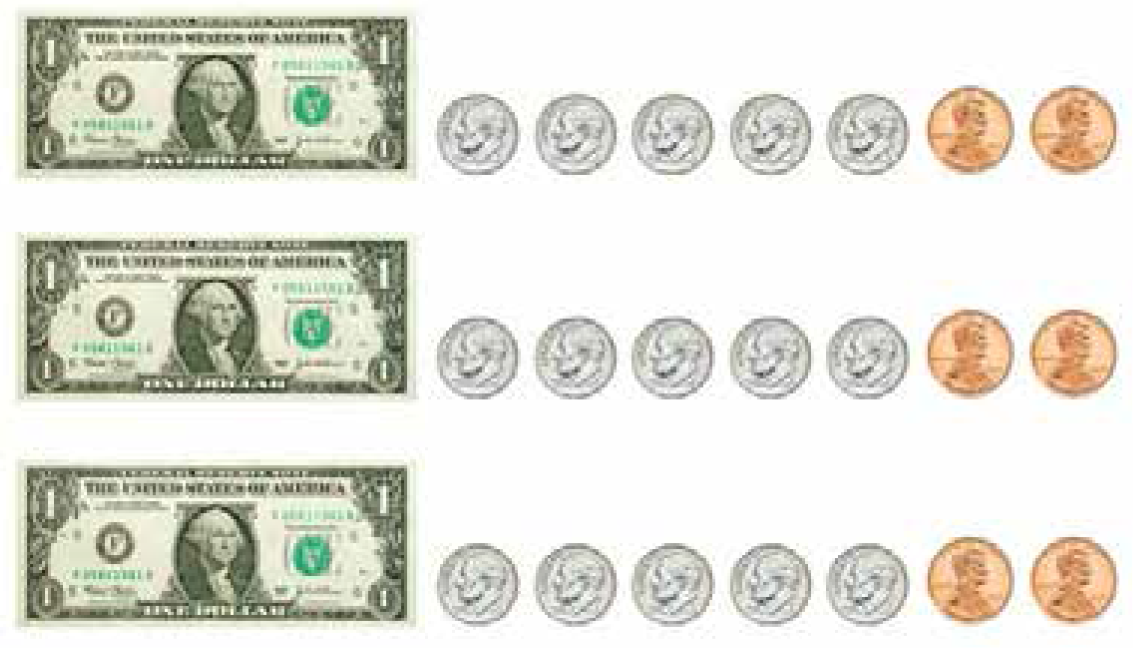Note that this picture is a correct, but awkward, way of computing and showing the new, tripled amount of money. Ask volunteers from groups working with their own money sets to represent the product (3 × 1.52) in a quicker, more compact way—for example, by using as many dollar bills and/or as few coins as possible. Methods of doing so (algorithms) may well vary; accept all responses that show the correct product in what- ever combinations of bills, dimes and pennies. Now present the logic of the standard algorithm, in pictorial terms.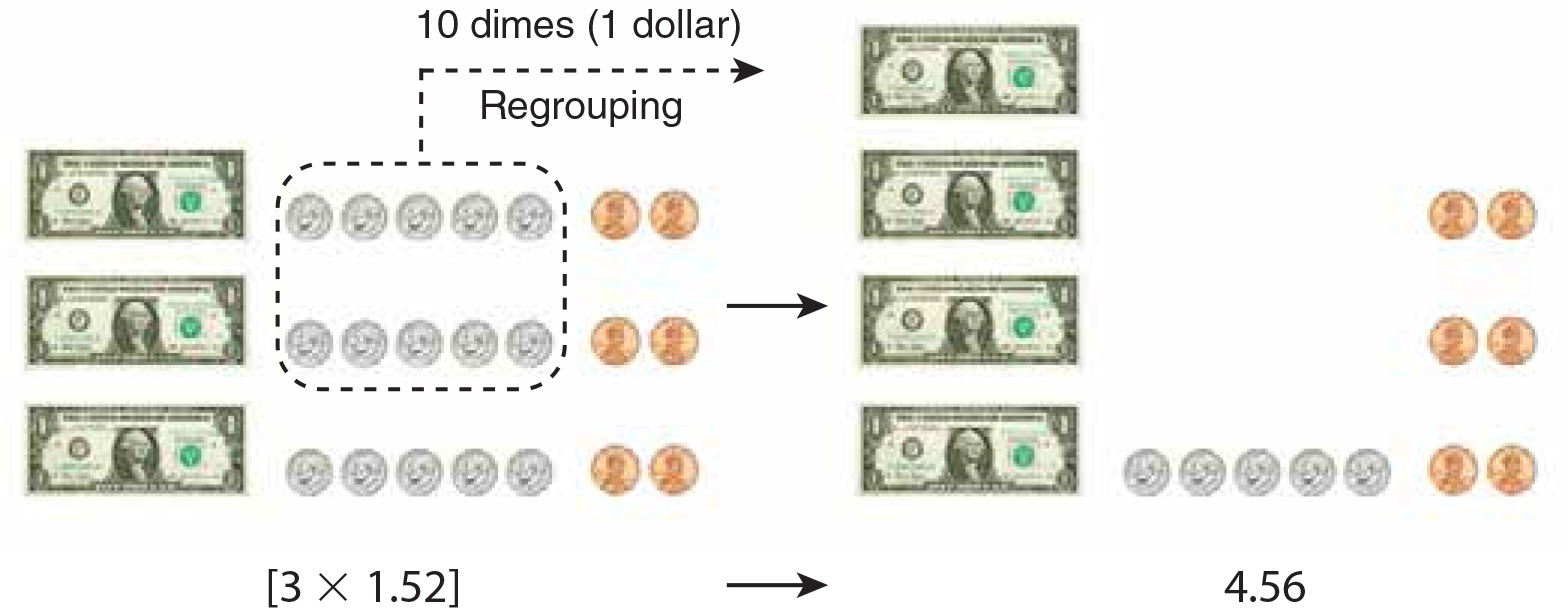Those students who did not produce this particular solution themselves are likely to agree that it is preferable to taking play money from their pockets or backpacks to figure out how much 3 cans of a beverage at \$1.52 a can will cost. Now have a volunteer represent the multiplication in the “mature” way by using a numerical procedure (symbolic algorithm). Be sure you and your class correlate each visual change in the money model with each step of this abstract procedure.Now, put the whole algorithm together.You may of course also use connecting cubes or base-10 blocks to carry out such demonstrations linking semantic and syntactical mathematical meanings.

References
“Multiplication and Division: From Whole Numbers to Rational Numbers”, A. O. Graeber and E. Tanenhaus, in Research Ideas for the Classroom, MacMillan/NCTM, 1993

## Simple Interest Modeled Concretely

### The interest formula is likely to be the first that students encounter with so many variables, and as such may cause confusion. To make each of the variable factors concrete, demonstrate using 1000-cubes, with which students may be familiar from modeling operations with mixed numbers and decimals. Pose a simple interest problem such as this to the class:

Al buys a used boat for \$1000. He has to pay 12% interest plus principal over 3 years. How much interest will Al end up paying?

Project images, or demonstrate concretely, to show the total amount of the loan and the part of the total that Al must pay in a year: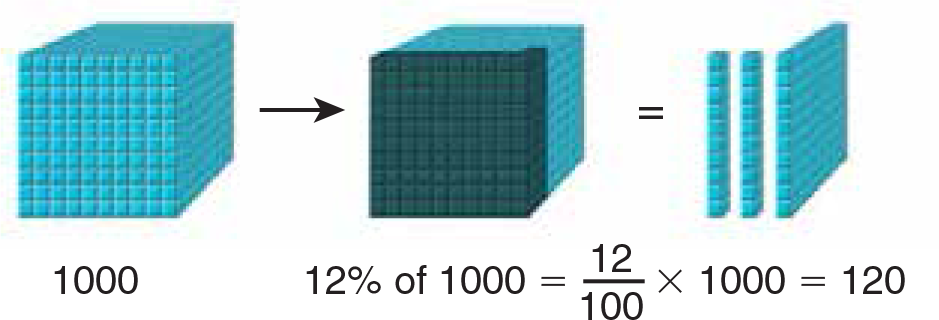Let students work in groups using 100-squares and 10-rods to show the part of the large cube that is 12%. Then ask if \$120 is all that Al has to pay in interest (no, he must pay the same for 3 years). Model (or have volunteers model) 3 years of interest at the same rate: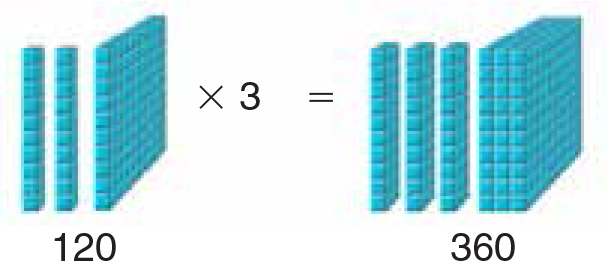At this point all 3 factors can be modeled together in one demonstration, using either the previous example, or another example, such as 8.2% on a \$1000 loan over 2 years: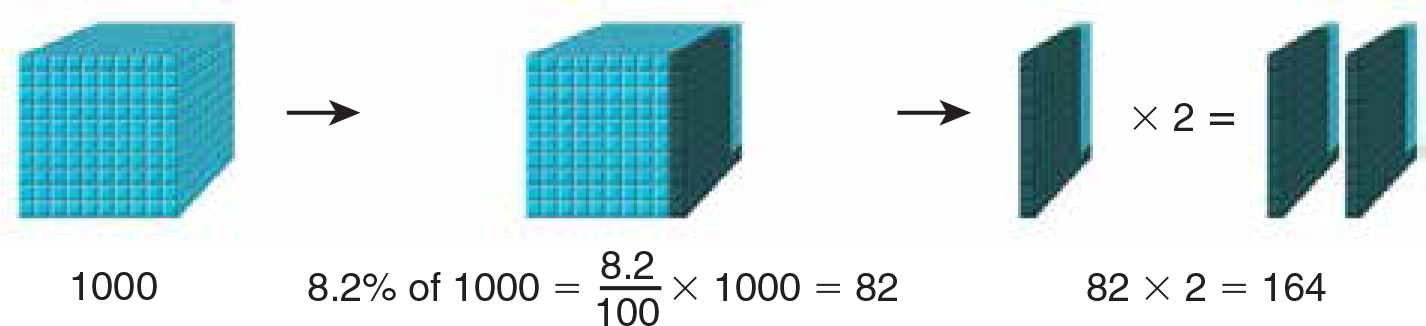Such a demonstration distinguishes and concretizes each of the 3 variable factors in the formula. Point out to the class that the same solutions to the problems can be found by multiplying together each of the 3 factors:and that the 3 factors in problems like this can be generalized into a formula:## Visual Estimates: Create Measurement Sense

### Applying formulas in geometry is an important aspect of the middle grades curriculum. But even many high school students are unsuccessful at using simple formulas. For such students, an abstract formula needs to be the outcome of guided exploration and, in particular, developed as a quick and common-sense way of summing up units. By starting again with estimating measures—without precision tools or abstract procedures—it is possible for students to regain the basic, intuitive understandings that underlie measurement, regardless of the attribute of measurement—area, length, or volume—or size of the unit.

Start with given objects, such as those pictured, and no assumption as to a unit for measuring them. For each object, have students pick a unit of length for which the object would have roughly 1 square unit of area. For example, ask:

This object has an area of about 1 square unit of a certain length.
What unit of length could I be thinking about?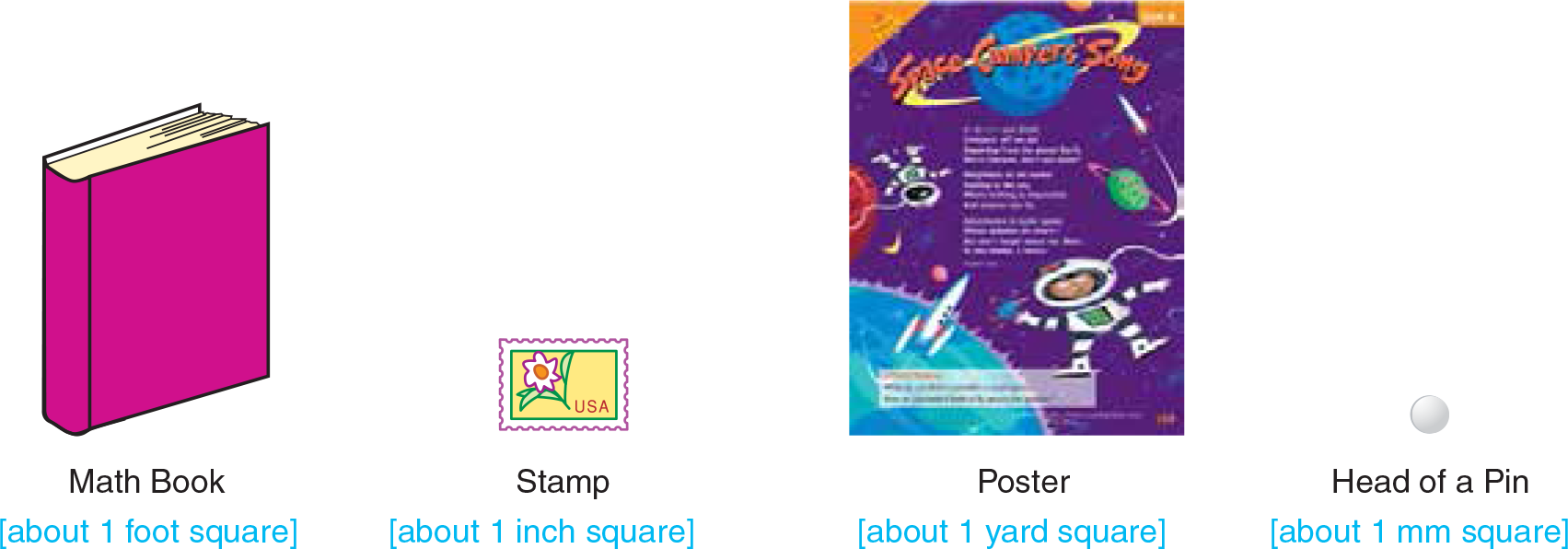Have students suggest and share their responses, without using pencil or paper (reason- able responses are given in brackets). After they provide appropriate answers, challenge them on the next level of understanding—that of the inverse relation of unit size to magnitude of measurement. Have students tell what happens to the size of the number describing these areas, when the unit is changed in size. For example:

• What happens to the area measurement of the book, if we use the unit inch instead of foot? Does the number for area become greater or less? (more) Why? (1 ft = 12 in., and 12 × 12 > 100, so area in inches is more)
• What happens to the area measurement of the book, if we use the unit yard instead of foot? (the measurement number gets smaller) Why? (1 ft = 13 yard, and 13 × 13110, so the area in yards is a fraction)

Have some students illustrate these understandings for the whole class: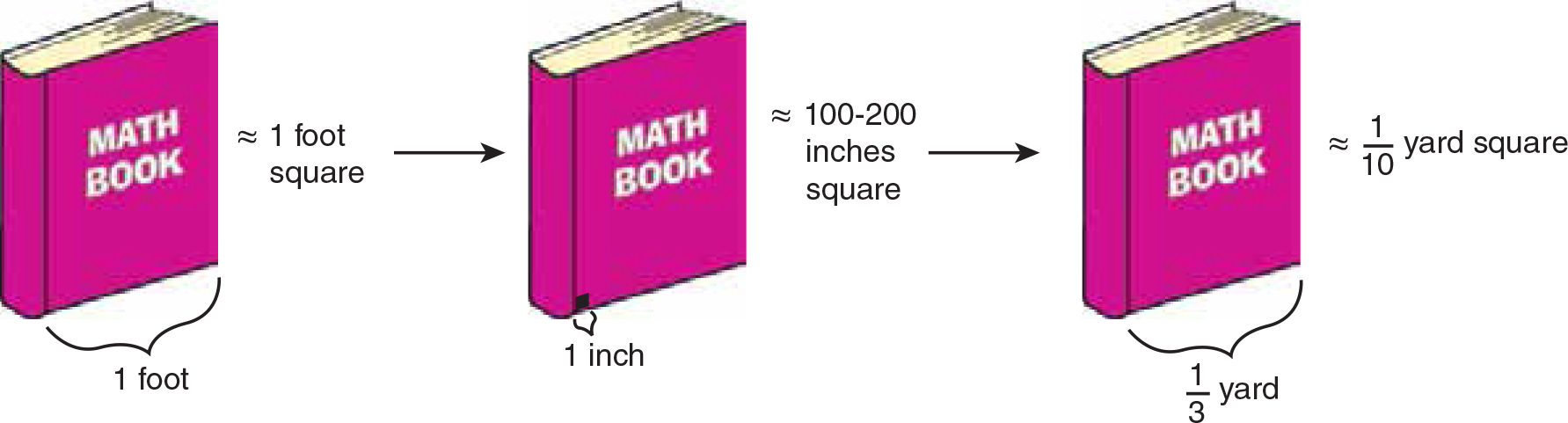The same reasoning process may be applied to other examples, with either simpler attributes of measure such as linear, or more complex ones such as volume, depending on the needs of students. The key ideas to aim for:

• the unit size and the numerical measurement result vary inversely
• formulas are ways of computing and recording measurements, provided the type of measure (length, area, or volume) and the unit are decided.